# GMAT Math : Calculating if two acute / obtuse triangles are similar

## Example Questions

### Example Question #1 : Calculating If Two Acute / Obtuse Triangles Are Similar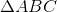is an equilateral triangle. Points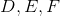are the midpoints of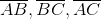, respectively.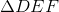is constructed.

All of the following are true except:

The perimeter ofis twice that of.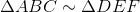Each side ofis parallel to a side of.

All of the statements in the other four choices are correct.

The area ofis twice that of.

The area ofis twice that of.

Explanation:

The three sides ofare the midsegments of, sois similar to.

By the Triangle Midsegment Theorem, each is parallel to one side ofBy the same theorem, each has length exactly half of that side, givingtwice the perimeter of.

But since the sides ofhave twice the length of those of, the area of, which varies directly as the square of a sidelength, must be four times that of.

The correct choice is the one that asserts that the area ofis twice that of.

### Example Question #2 : Calculating If Two Acute / Obtuse Triangles Are Similar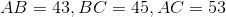.

Order the angles offrom least to greatest measure.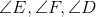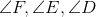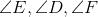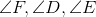The angles ofcannot be ordered from the information given.Explanation:

In a triangle, the angle of greatest measure is opposite the side of greatest measure, and the angle of least measure is opposite the side of least measure.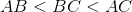, so their opposite angles are ranked in this order - that is,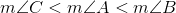.

Corresponding angles of similar triangles are congruent, so, since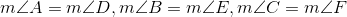.

Therefore, by substitution,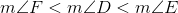.

### Example Question #3 : Calculating If Two Acute / Obtuse Triangles Are Similar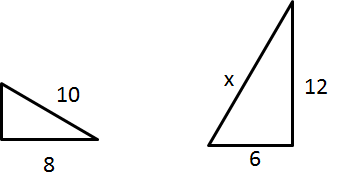The triangles are similar. What is the value of x?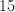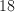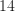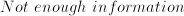The proportions of corresponding sides of similar triangles must be equal. Therefore,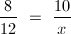.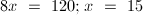.